# Introduction

Subsets play an important role in almost any data analysis. Suppose we have a dataset of countries, and our main interest is countries in Africa. Further, suppose we wish to examine nested subsets such as (1) countries in Africa, (2) with populations exceeding 50 million, (3) that are landlocked. In this situation, we might ask questions like:

• Of African countries with populations not exceeding 50 million, what proportion are landlocked?

• What is the average GDP in African countries with populations over 50 million?

Even in a simple situations like this, it can be a chore to keep track of nested subsets. The presence of missing values in data sets further complicates matters. And as additional subsets are examined, the magnitude of the task grows rapidly.

One way to represent nested subsets of a dataset is to use a tree structure, which we can call a variable tree. `vtree` is a flexible tool for drawing variable trees.

## Basic features of a variable tree

The examples that follow use a dataset of 46 fictitious patients called `FakeData`. Based on this dataset, the variable tree below depicts subsets defined by `Sex` (M or F) nested within subsets defined by disease `Severity` (Mild, Moderate, Severe, or NA).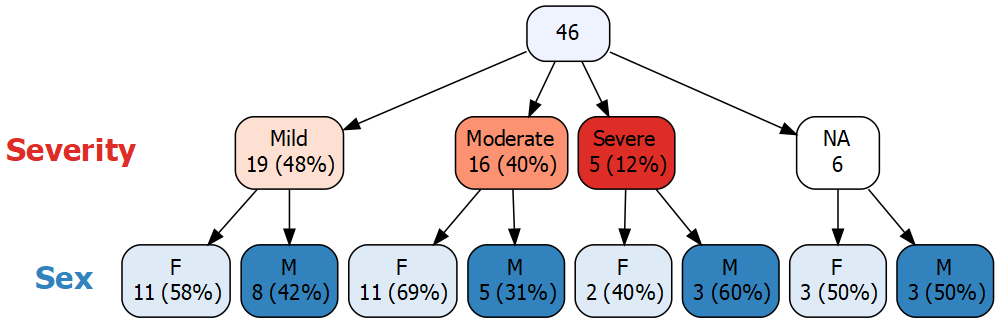A variable tree consists of nodes connected by arrows. At the top of the diagram above, the root node of the tree contains all 46 patients. The rest of the nodes are arranged in successive levels, where each level corresponds to a variable. The nodes immediately below the root represent values of `Severity` and are referred to as the children of the root node. In this case, `Severity` was missing (NA) for 6 patients, and there is a node for these patients. Inside each of the nodes, the number of patients is displayed and—except for the missing value node—the corresponding percentage is also shown. Note that, by default, `vtree` displays “valid” percentages, i.e. the denominator used to calculate the percentage is the total number of non-missing values, 40.

The nodes in the next level (which is the final level for this tree) correspond to values of `Sex`. These nodes represent males and females within subsets defined by each value of `Severity`. In each of these nodes the percentage is calculated in terms of the number of patients in its parent node.

Like any node, a missing-value node can have children. For example, of the 6 patients for whom `Severity` is missing, 3 are female and 3 are male. By default, `vtree` displays the full missing-value structure of the specified variables in the data frame.

Also by default, `vtree` automatically assigns a color palette to each variable. `Severity` has been assigned red hues (lightest for Mild, darkest for Severe), while `Sex` has been assigned blue hues (light blue for females, dark blue for males). The node representing missing values of `Severity` is colored white to draw attention to it.

## Applications of variable trees

A tree with two variables is equivalent to a two-way contingency table with either row or column percentages, depending on which variable comes first in the tree. In the example above, `Sex` is shown within levels of `Severity`. This corresponds to the following contingency table, with column percentages (i.e., percentages within each column add to 100%).

Mild Moderate Severe NA
F 11 (58%) 11 (69%) 2 (40%) 3 (50%)
M 8 (42%) 5 (31%) 3 (60%) 3 (50%)

Variable trees are easy to interpret because they represent subsets visually. Contingency tables can be harder to interpret, especially when they involve more than two variables.

Variable trees are thus a convenient alternative to multi-way contingency tables and can also be used to display a wide variety of information including:

• multi-way intersections (often shown in Venn diagrams),

• flow diagrams involving a sequence of inclusion/exclusion steps,

• longitudinal events.

## Features of vtree

`vtree` is designed to be quick and easy to use, so that it is convenient for data exploration, but also flexible enough that it can be used to prepare publication-ready figures.

To make variable trees easier to interpret, `vtree` supports custom labeling of variables and nodes. One challenge with variable trees is that as variables are added, trees can get very large. For this reason, `vtree` includes tools for pruning. Variable trees can also be used to display additional subset-specific information. For example, suppose you wished to know the mean age within each subset defined by the specified variables. `vtree` makes it easy to display such information in each node. Finally, `vtree` supports numerous additional customizations and formatting tweaks.

To summarize, `vtree` implements several additional features:

• flexible pruning to remove parts of the tree that are of lesser interest

• display of summary statistics for other variables (e.g. continuous variables) in each node

• renaming of variables and nodes

• additional customization and formatting options

## Technical overview

`vtree` is built on open-source software: in particular Richard Iannone’s DiagrammeR package, which provides an interface to the Graphviz software using the htmlwidgets] framework. A formal description of variable trees follows.

The root node of the variable tree represents the entire data frame. The root node has a child for each observed value of the first variable that was specified. Each of these child nodes represents a subset of the data frame with a specific value of the variable, and is labeled with the number of observations in the subset and the corresponding percentage of the number of observations in the entire data frame. The nth level below the root of the variable tree corresponds to the nth variable specified. Apart from the root node, each node in the variable tree represents the subset of its parent defined by a specific observed value of the variable at that level of the tree, and is labeled with the number of observations in that subset and the corresponding percentage of the number of observations in its parent node.

Note that a node always represents at least one observation. Unlike a contingency table, which can have empty cells, a variable tree has no empty nodes.

# The `vtree` function

Consider a data frame named `df`, which includes categorical variables `v1` and `v2`. In this case, a variable tree can be displayed using the following command:

``vtree(df,"v1 v2")``

For convenience, `vtree` allows you to specify the variable names in a single character string (with the variable names separated by whitespace). If, however, any of the variable names have internal spaces, the variable names must be specified as a vector of character strings.

Numerous additional parameters can be supplied. For example, by default `vtree` produces a horizontal tree (that is, a tree that grows from left to right), but sometimes a vertical tree is preferable. When `horiz=FALSE` is specified, `vtree` generates a vertical tree.

## Mini tutorial

To display a variable tree for a single variable, use the following command:

``vtree(FakeData,"Severity")``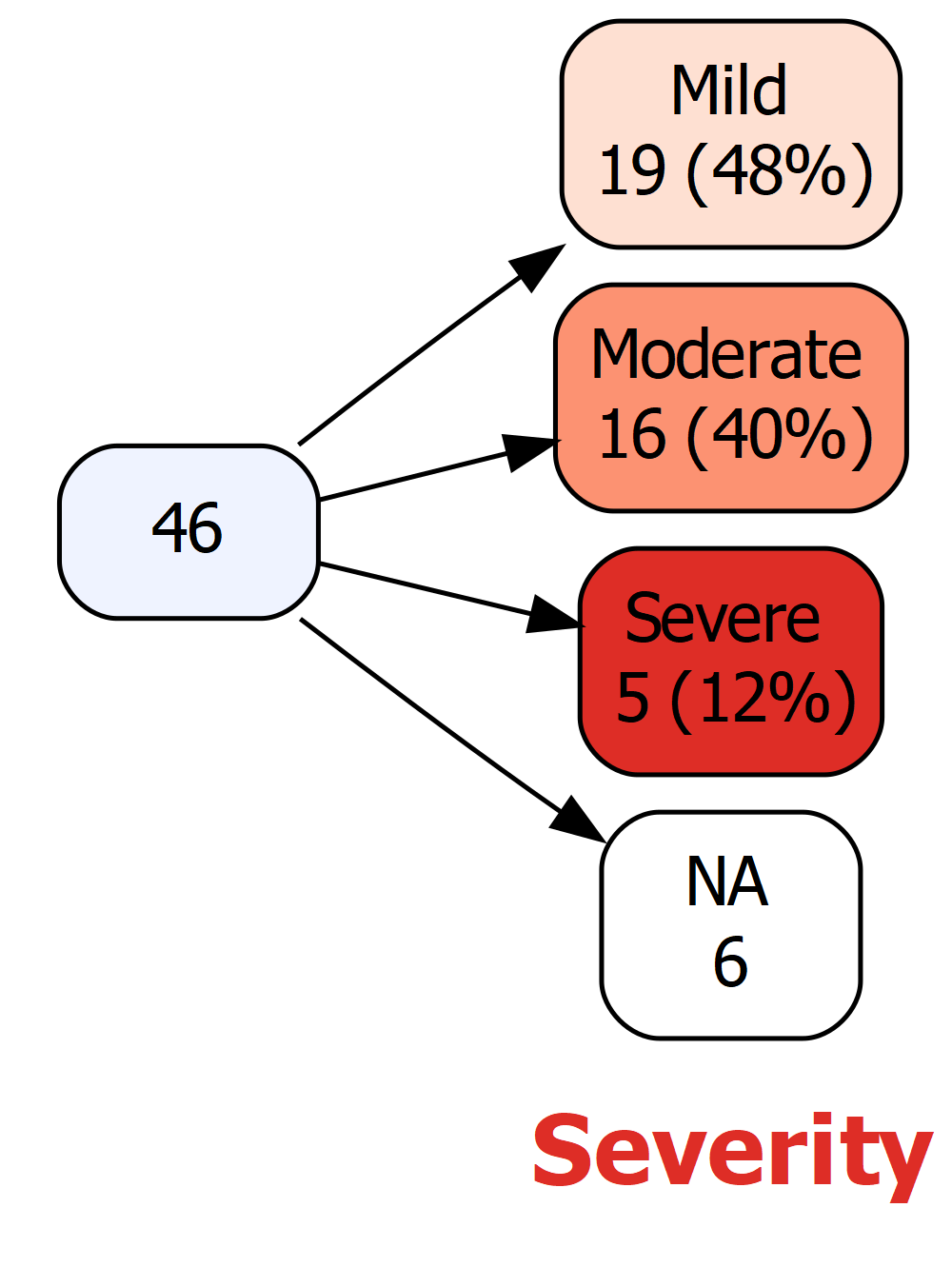Now here’s a vertical variable tree with two variables, `Severity` and `Sex`. A less colorful display with more spacing has been requested by specifying `plain=TRUE`:

``vtree(FakeData,"Severity Sex",horiz=FALSE,plain=TRUE)``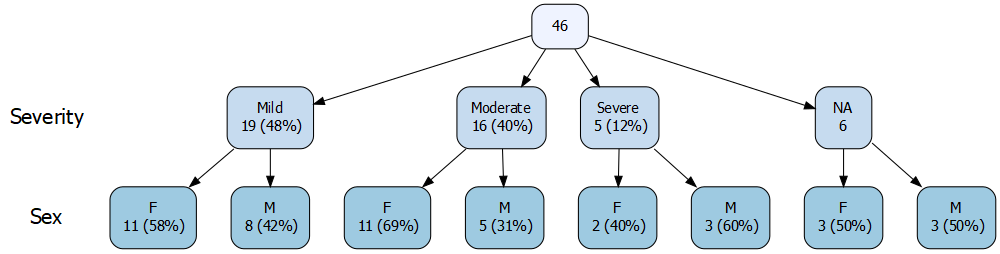At the top, the root node represents the entire data frame. Moving down, each subsequent level of the tree corresponds to a different variable (first `Severity`, then `Sex`). Within each level, each node represents the subset of its parent node where the variable has a specific value. For example, the level for `Severity` has nodes Mild, Moderate, Severe, and NA (which represents missing values). Displayed in each node is the number of observations and (except in the NA node) the conditional percentage, i.e. the number of observations in the node expressed as a percentage of the observations in its parent node.

### Percentages

By default, “valid percentages” are shown, i.e. the denominator is the total number of non-missing values. In the case of `Severity`, there are 6 missing values, so the denominator is 46 - 6 , or 40. There are 19 Mild cases, and 19/40 = 0.475 so the percentage shown is 48%. No percentage is shown in the NA nodes since they are not included.

Alternatively, if you don’t want to use valid percentages, specify `vp=FALSE`, and the denominator will be the total number of observations, including any missing values. In this case, a percentage is shown in all of the nodes, including nodes for missing values.

If you don’t wish to see percentages, specify `showpct=FALSE`, or if you don’t need to see counts, specify `showcount=FALSE`.

### Displaying a legend and hiding node labels

To include a legend, specify `showlegend=TRUE`. Next to each level of the tree, the variable name is displayed together with color discs and the values they correspond to. For each of the values, overall (marginal) counts are shown, together with percentages.

When the legend is shown, the node labels become redundant, since the colors identify the values of the variables (although the labels may aid readability). If you prefer, you can hide the node labels, by specifying `shownodelabels=FALSE`:

``vtree(FakeData,"Severity Sex",showlegend=TRUE,shownodelabels=FALSE)``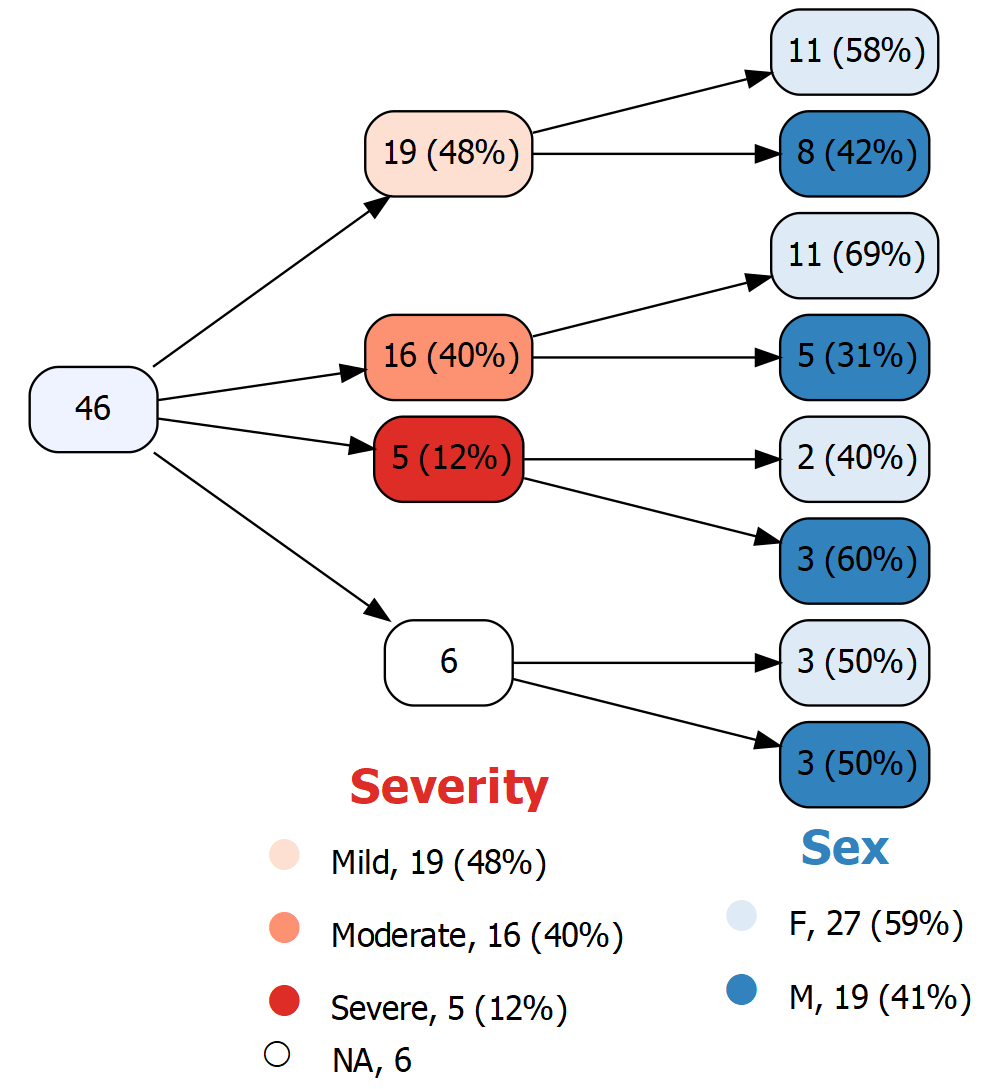The legend shows how colors are assigned to the different values of each variable, and additionally provides marginal (that is, overall) counts and percentages for each variable. Since `Severity` is the first variable in the tree—i.e., it is not nested within another variable— the marginal counts and percentages for `Severity` are identical to those displayed in the nodes. In contrast, for `Sex`, the marginal counts and percentages are different from what is shown in the nodes because the nodes for `Sex` are nested with levels of `Severity`.

(Unfortunately the NA disc in the legend is oddly sized and positioned due to an issue with the corresponding unicode symbols.)

### Putting node labels on the same line as the counts and percentages

When a variable tree is large, it can be difficult to display clearly. One approach is to display it horizontally and also to put the node labels on the same line as the counts and percentage by specifying `sameline=TRUE`. For example, the following results in nodes labeled Moderate, 16 (40%) etc:

``vtree(FakeData,"Severity Sex Viral",sameline=TRUE)``

### Hiding variable names

By default, `vtree` shows the variable names next to the corresponding levels of the tree. These can be removed by specifying `showvarnames=FALSE`.

### Text wrapping

By default, `vtree` wraps text onto the next line whenever a space occurs after at least 20 characters. This can be adjusted, for example, to 15 characters, by specifying `splitwidth=15`. Text wrapping in the legend is controlled independently. To set the splitting in the legend to 8 characters, specify `lsplitwidth=8`.

## Pruning

Pruning a tree means removing specified nodes (and their descendants). This is useful when a variable tree gets too big, or when you are only interested in certain parts of the tree.

### The `prune` parameter

Suppose you don’t want the tree to include individuals whose disease is Mild or Moderate. You can use the `prune` parameter to remove those nodes, and all of their descendants.

The `prune` parameter is specified as a list with an element named for each variable you wish to prune. In the example below the list has one element, named `Severity`. That element in turn is a vector `c("Mild","Moderate")` indicating the values to prune.

``vtree(FakeData,"Severity Sex",prune=list(Severity=c("Mild","Moderate")))``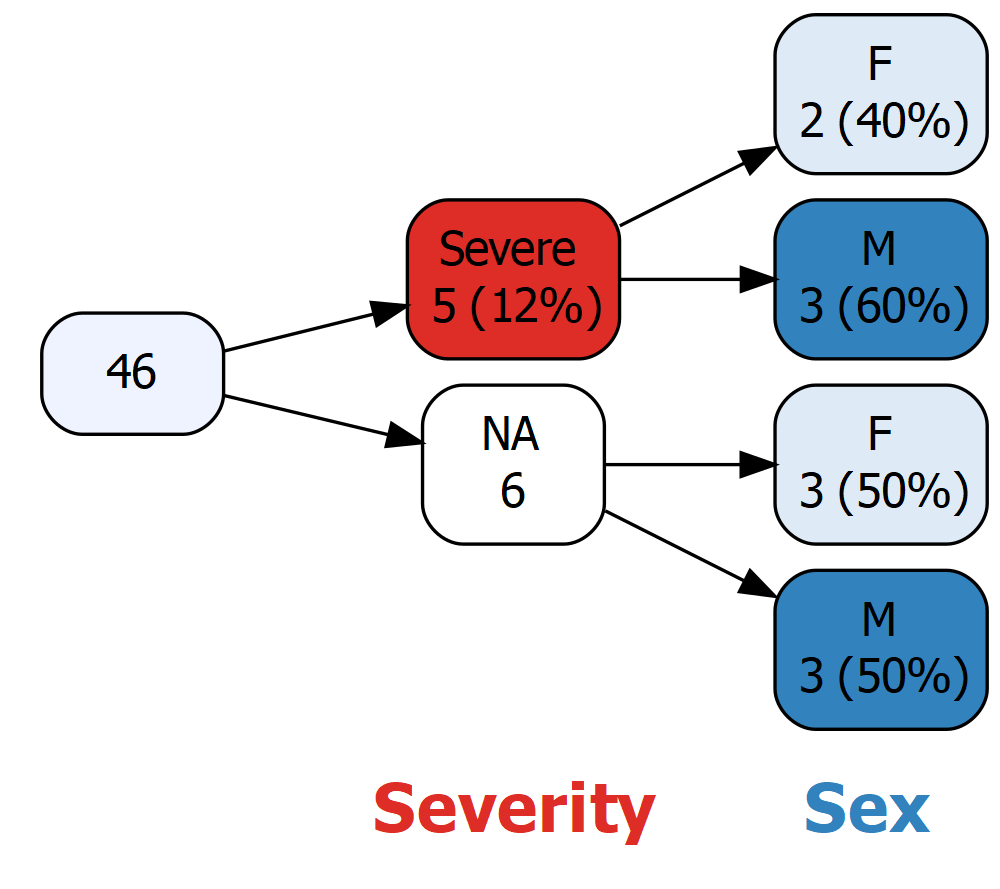Caution: Once a variable tree has been pruned, it is no longer complete. This can sometimes be confusing since not all observations are present at some levels of the tree. It is particularly important to avoid pruning missing value nodes, since this makes it hard to interpret “valid” percentages (i.e. percentages calculated using the number of non-missing observations as denominator).

### The `prunebelow` parameter

The `prune` parameter completely eliminates nodes (along with their descendants). A disadvantage of this is that the counts shown in child nodes do not add up to the counts shown in the parent node. For example in the variable tree above, of a total of 46 patients, 5 have Severe disease and `Severity` is unknown for 6. One might wonder what happened to the other 35 patients.

An alternative is to prune below the specified nodes. In this case, this means that the Mild and Moderate nodes will be shown, but not their descendants.

``vtree(FakeData,"Severity Sex",prunebelow=list(Severity=c("Mild","Moderate")))``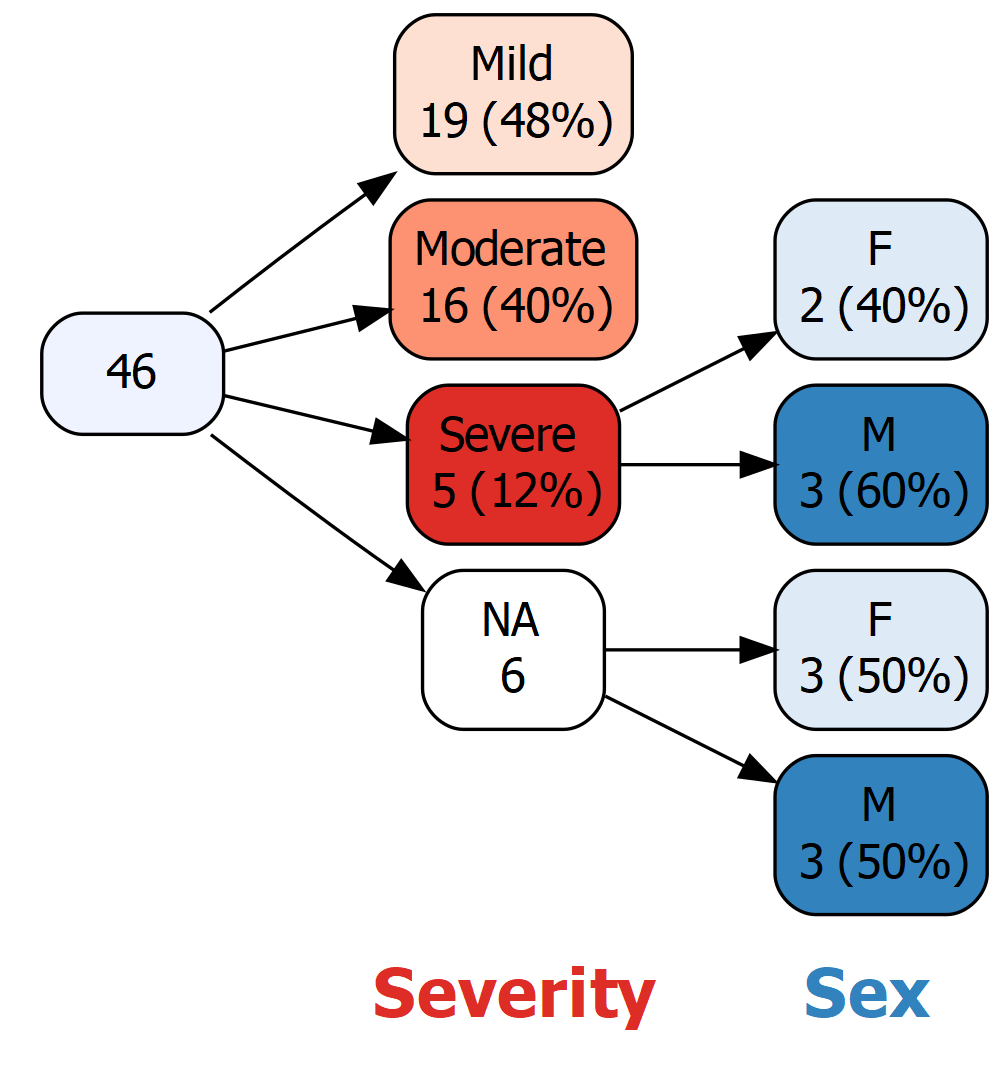### The `keep` and `follow` parameters

Instead of specifying the nodes that should be discarded, sometimes it is more convenient to specify the nodes that should be retained. The `keep` parameter is used to specify nodes that should not be pruned (all other nodes at that level of the tree will be pruned). The `follow` parameter is like the `keep` parameter except that no nodes at that level of the tree will be pruned. Instead, those nodes that are not “followed” will be pruned at the next level.

## Renaming nodes and variables

It’s often useful to specify a more informative label in place of the variable name. For example, if `Severity` in fact represents severity on day 1, you might want it to appear that way in the variable tree. To do this, use the `labelvar` parameter, which is specified as a vector whose element names are variable names. As an example, if `Severity` in fact represents severity on day 1, you can specify `labelvar=c(Severity="Severity on day 1")`.

By default, `vtree` names nodes (except for the root node) using the values of the variable in question. (If the variable is a factor, the levels of the factor are used). Sometimes it is convenient to instead specify custom labels for nodes. You can use the `labelnode` argument to relabel the values. For example, you might want to use “Male” and “Female” instead of “M” and “F”. The `labelnode` argument argument is specified as a list whose element names are variable names. To substitute `New label` for `Old label`, the syntax is: `"New label"="Old label"`. Thus the full specification is: `labelnode=list(Sex=c(Male="M",Female="F"))`.

``````vtree(FakeData,"Severity Sex",horiz=FALSE,
labelvar=c(Severity="Severity on day 1"),labelnode=list(Sex=c(Male="M",Female="F")))``````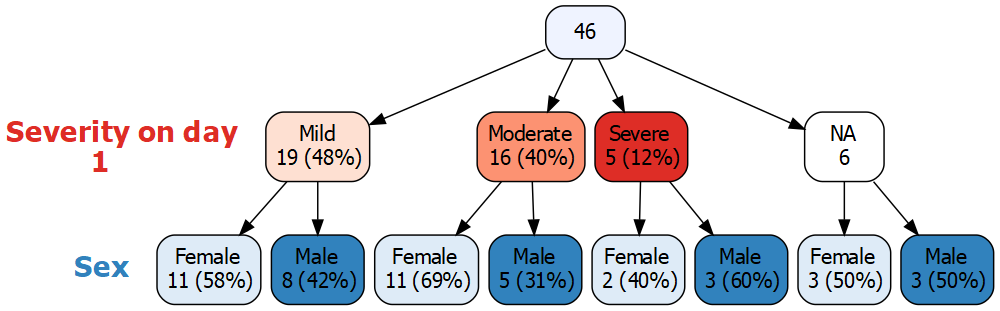## Text and text formatting

`Graphviz`, the open source graph visualization software that provides the basis for `vtree`, supports a variety of text formatting (including boldface, colors, etc.). This is used in `vtree` to control formatting of text such as node labels.

### HTML-style codes for text formatting

NOTE: The section after this one shows how to use an easy alternative to HTML-style codes.

`Graphviz` implements “HTML-style” codes, including:

• `<BR/>` means insert a line break
• `<BR ALIGN='LEFT'/>` means make the preceding line left-justified and insert a line break
• `<I> ... </I>` means display text in italics
• `<B> ... </B>` means display text in bold
• `<SUP> ... </SUP>` means display text in superscript, but note that the font size does not change
• `<SUB> ... </SUB>` means display text in subscript but again note that the font size does not change
• `<FONT POINT-SIZE='10'> ... </FONT>` means set font to 10 point
• `<FONT FACE='Times-Roman'> ... </FONT>` means set font to Times-Roman
• `<FONT COLOR='red'> ... </FONT>` means set font to red

See https://www.graphviz.org/doc/info/shapes.html#html for more details.

Note: To use these HTML-style codes, it is necessary to specify `HTMLtext=TRUE`.

### Markdown-style codes for text formatting

By default, the `vtree` package uses markdown-style codes for text formatting.

• `\n` means insert a line break
• `\n*l` means make the preceding line left-justified and insert a line break
• `*...*` means display text in italics
• `**...**` means display text in bold
• `^...^` means display text in superscript (using 10 point font)
• `~...~` means display text in subscript (using 10 point font)
• `%%red ...%%` means display text in red (or whichever color is specified)

### Adding text to nodes using the `text` parameter

Suppose you wish to add the italicized text “Includes first-time visits” to the Mild node. The parameter `text` lets you add text to nodes. It is specified as a list with an element named for each variable. In the example below the list has one element, named `Severity`. That element in turn is a vector `c(Mild="*Includes first-time visits*")` indicating that the Mild node should include additional text using Markdown-style formatting (i.e. the asterisks around the text indicate that it should be displayed in italics):

``````vtree(FakeData,"Severity",horiz=FALSE,
text=list(Severity=c(Mild="*Includes first-time visits*")))``````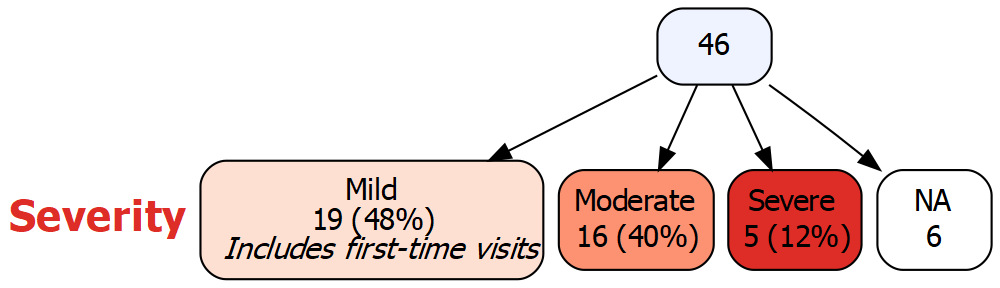## Displaying summary statistics in nodes

It is often useful to display information about other variables (apart from those that define the tree) in the nodes of a variable tree. For example, we might wish to display the mean age for individuals in each node. Or we might wish to list the ID numbers for individuals in each node. The `summary` argument can be used to flexibly specify additional information to display.

### A simple example

The `summary` parameter is specified as a character string that starts with the variable in question. This is followed by a space, and then the rest of the string specifies what kind of summary to display. Special codes are use to indicate the type of summary desired. For example, `%mean%` is used to specify that the mean of the variable should be displayed.

For example, to display the mean of the variable `Score`, specify `summary="Score %mean%"`:

``vtree(FakeData,"Severity",summary="Score %mean%",horiz=FALSE)``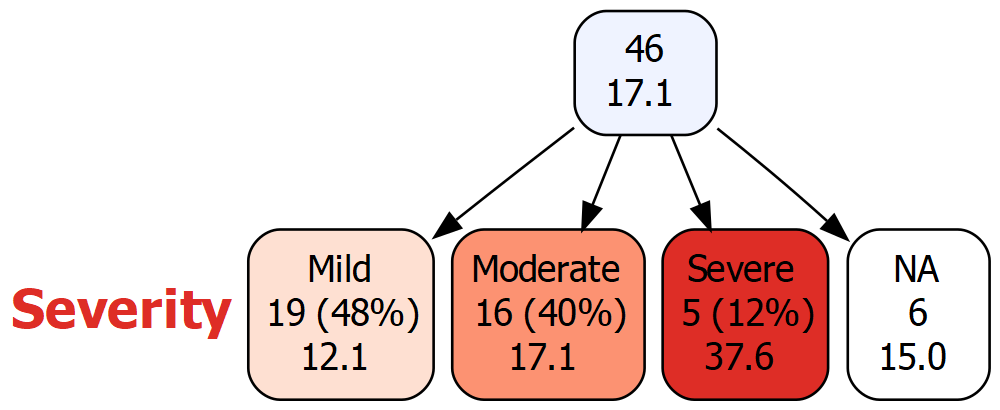The following summary codes can be used by `summary`:

• `%mean%` mean
• `%SD%` standard deviation
• `%min%` minimum
• `%max%` maximum
• `%pX%` Xth percentile (e.g. `p50` means the 50th percentile)
• `%median%` median, i.e. p50
• `%IQR%` IQR, i.e. p25, p75
• `%npct%` n (%). By default “valid percentages” are used. Any missing values are also reported.
• `%list%` list of the individual values
• `%mv%` the number of missing values
• `%v%` the name of the variable
• `%noroot%` flag: Do not show summary in the root node.
• `%leafonly%` flag: Only show summary in leaf nodes, i.e. nodes that have no children.
• `%node=`n`%` flag: Only show summary in the specified node.
• `%trunc=`n`%` flag: Truncate the summary to the first n characters.

The `summary` argument can use any number of these codes, mixed with text and formatting codes.

### More than one variable

Sometimes it is useful to display summary information for more than one variable. To do this, specify `summary` as a vector of character strings:

``````vtree(FakeData,"Severity",horiz=FALSE,showvarnames=FALSE,
summary=c(
"Score \nScore: mean (SD)\n %mean% (%SD%)",
"Pre \n\nPre: range\n %min%, %max%"))``````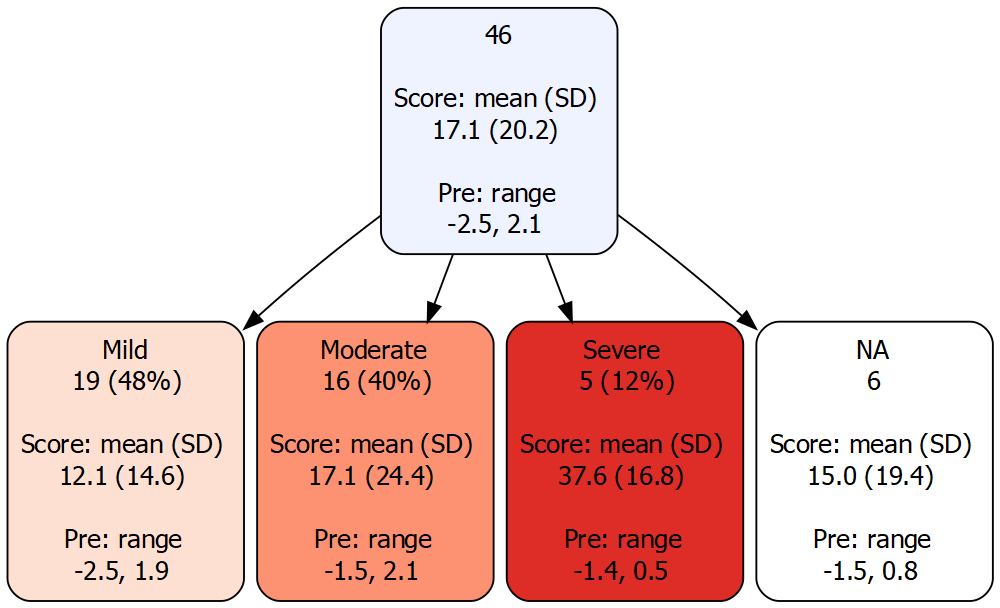### The %npct% code

Suppose we want to know, within each severity level, what proportion of patients have a viral infection. We could display a variable tree for the variables `Severity` and `Viral`. But that would show a separate node for TRUE and FALSE values of `Viral`, and we don’t need to examine these subsets. If what we’re looking for is simply the number and percentage of patients with viral infection in each severity group, the `%npct%` code can be used. This results in a simpler tree:

``vtree(FakeData,"Severity",summary="Viral \nViral %npct%",horiz=FALSE,showvarnames=FALSE)``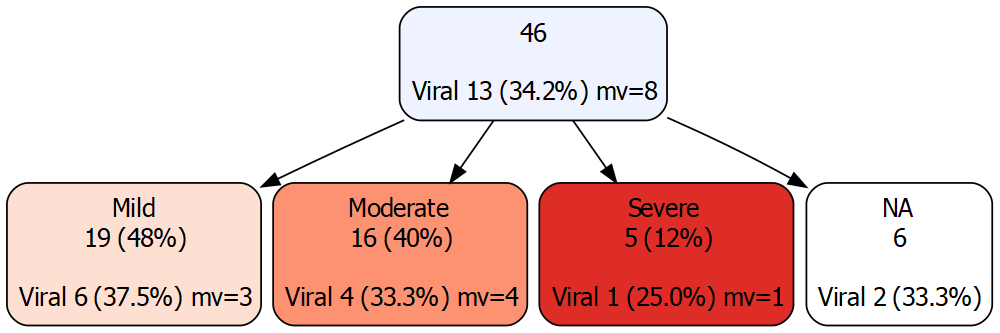Note that in each node, “mv” indicates the number of missing values (if any).

### The %list% code

It is sometimes convenient to see individual values of a variable in each node. For example it is often convenient to see ID numbers. To do this, use the `%list%` code. By default this information will be displayed in each node. It may also be convenient to only show the information in certain nodes. For example we might only want to see the information in the leaf nodes.

In this simple case the following codes are equivalent:

• `%noroot%`

• `%leafonly%`

• `%node=Severity%`

When there are many IDs, it often convenience to truncate the output. The `%trunc=N%` code specifies that, after N characters, summary information should be truncated with “…”.

For example,

``````vtree(FakeData,"Severity",summary="id \nid = %list% %node=Severity% %trunc=40%",
horiz=FALSE,showvarnames=FALSE)``````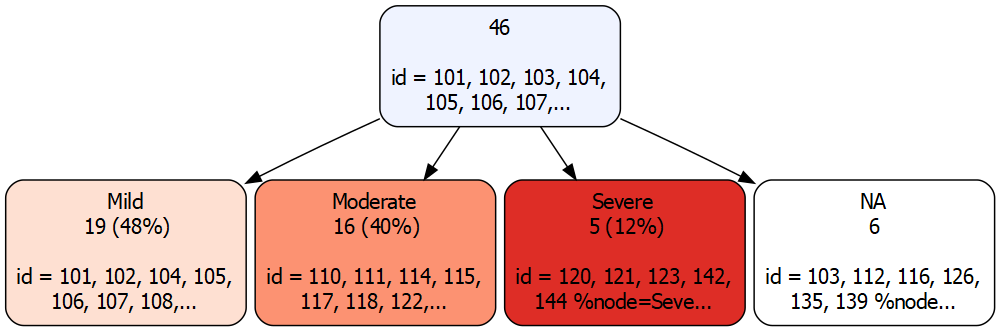## Examining the DOT script generated by `vtree`

Specifying `getscript=TRUE` lets you capture the DOT script representing a flowchart. Here is an example:

``````dotscript <- vtree(FakeData,"Severity",getscript=TRUE)
cat(dotscript)``````
``````digraph vtree {
graph [layout = dot, compound=true, nodesep=0.1, ranksep=0.5, fontsize=12]
node [fontname = Helvetica, fontcolor = black,shape = rectangle, color = black,margin=0.1]
rankdir=LR;
Node_L0[style=invisible]
Node_L1[label=<<FONT POINT-SIZE="20"><FONT COLOR="#DE2D26"><B>Severity  </B></FONT></FONT><BR/>> shape=none margin=0]

edge[style=invis];
Node_L0->Node_L1

edge[style=solid]
Node_1->Node_2 Node_1->Node_3 Node_1->Node_4 Node_1->Node_5

Node_1[label=<46> color=black style="rounded,filled" fillcolor=<#EFF3FF>]
Node_2[label=<Mild<BR/>19 (48%)> color=black style="rounded,filled" fillcolor=<#FEE0D2>]
Node_1[label=<46> color=black style="rounded,filled" fillcolor=<#EFF3FF>]
Node_3[label=<Moderate<BR/>16 (40%)> color=black style="rounded,filled" fillcolor=<#FC9272>]
Node_1[label=<46> color=black style="rounded,filled" fillcolor=<#EFF3FF>]
Node_4[label=<Severe<BR/>5 (12%)> color=black style="rounded,filled" fillcolor=<#DE2D26>]
Node_1[label=<46> color=black style="rounded,filled" fillcolor=<#EFF3FF>]
Node_5[label=<NA<BR/>6> color=black style="rounded,filled" fillcolor=<white>]

}``````

If you wish to directly edit this code, it can can be pasted into one of these online Graphviz editors:

https://dreampuf.github.io/GraphvizOnline

http://magjac.com/graphviz-visual-editor/

## Special variable trees

### Multi-way intersections (often shown in Venn diagrams)

A Venn diagram is defined by a set of variables that indicate whether an observation belongs to each of several sets. When there are more than three sets, Venn diagrams tend to be hard to read. Additionally, Venn diagrams cannot represent missing values.

Variable trees provide an alternative. In the following example, the variables `Ind1` through `Ind4` are indicators of set membership (0 = not a member of the set, 1 = member). Convenient settings for such variables are requested by specifying `Venn=TRUE`:

``vtree(FakeData,"Ind1 Ind2 Ind3 Ind4",Venn=TRUE)``